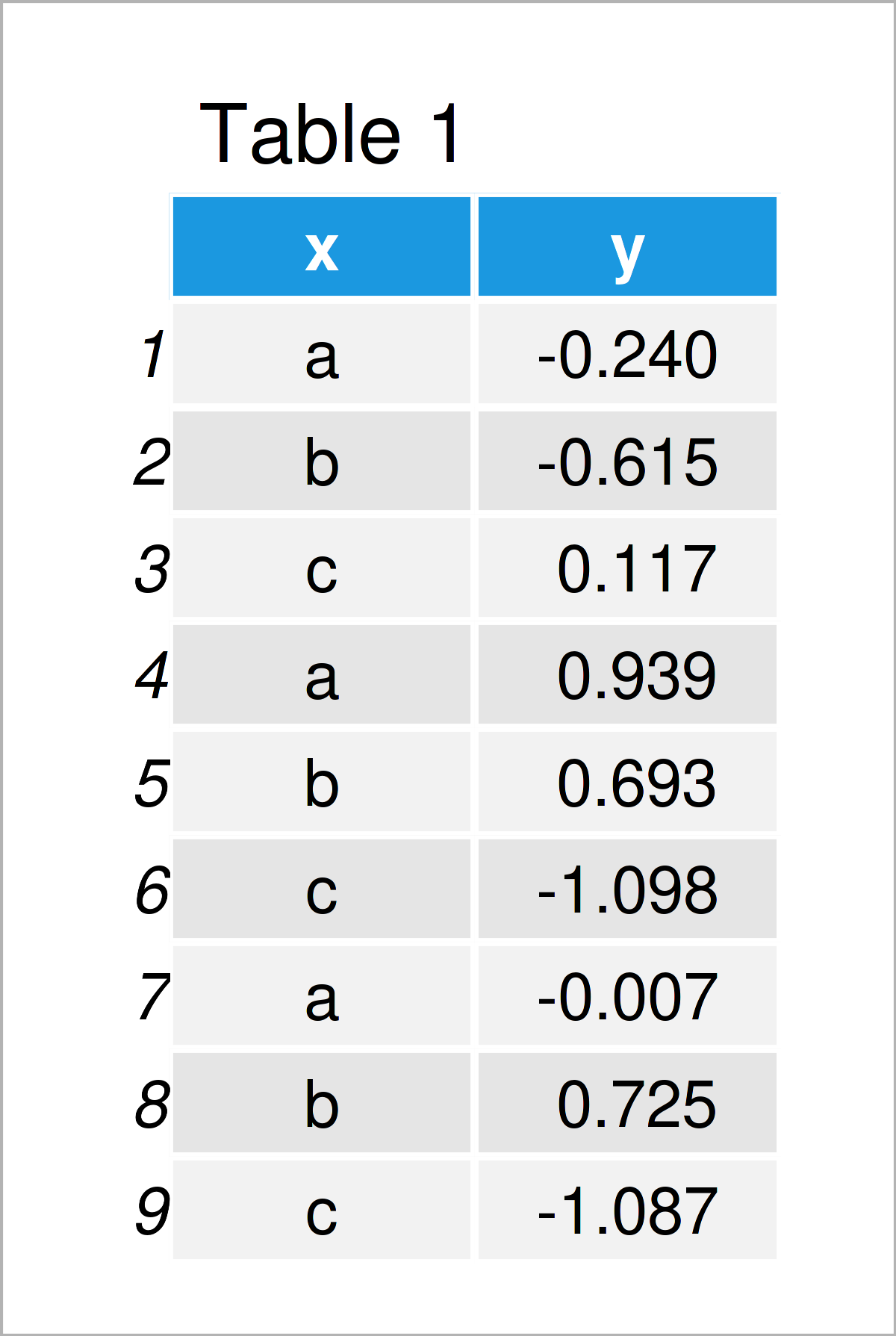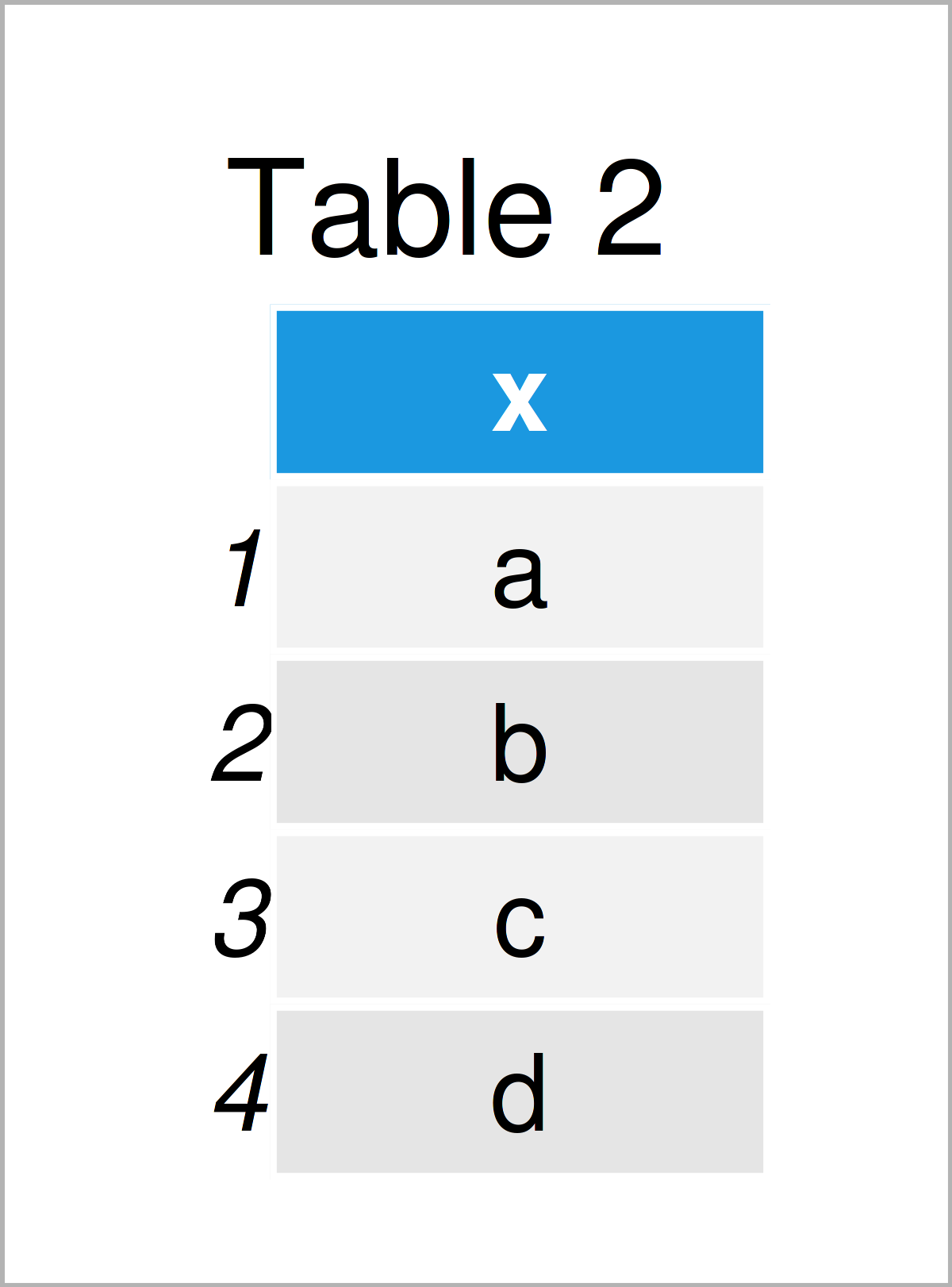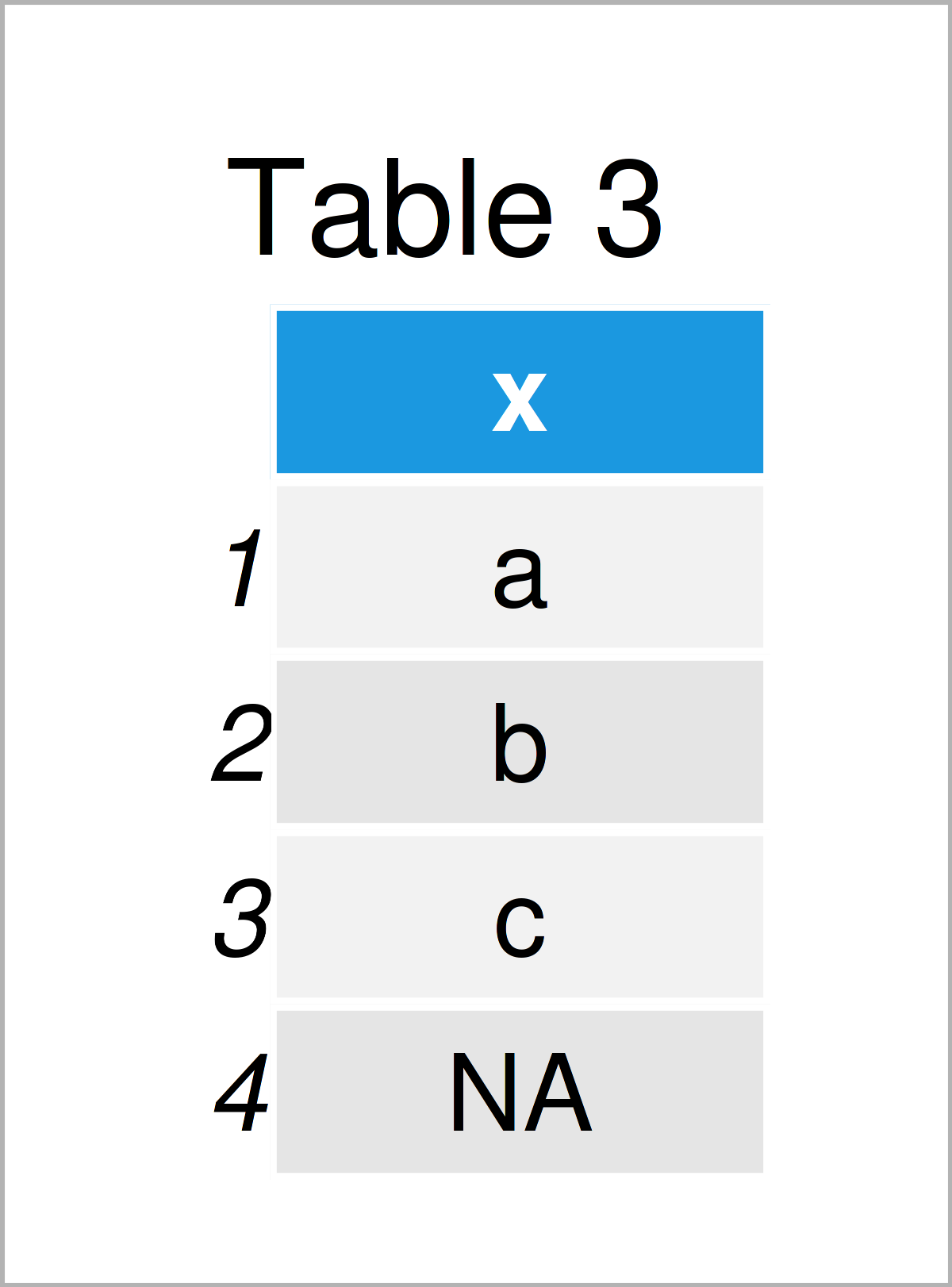# R Error in model.frame.default(Terms, newdata, na.action = na.action, xlev = object\$xlevels) : factor X has new levels Y

In this tutorial, I’ll explain how to reproduce and fix the “Error in model.frame.default(Terms, newdata, na.action = na.action, xlev = object\$xlevels) : factor X has new levels Y” in the R programming language.

Let’s dig in…

## Creation of Example Data

At the start, let’s construct some example train data for our linear regression model:

```set.seed(54136278)                                        # Set random seed
data_train <- data.frame(x = letters[1:3],                # Create train data set
y = rnorm(9))
data_train                                                # Print train data set```Table 1 shows the structure of our example data: It consists of nine rows and two columns. The variable x is a character that will be used as a predictor variable. The variable y is numerical and will be used as the target variable.

Let’s conduct a linear regression based on our data:

```my_mod <- lm(y ~ x, data_train)                           # Estimate linear regression model
summary(my_mod)                                           # Summary statistics of regression model
# Call:
# lm(formula = y ~ x, data = data_train)
#
# Residuals:
#     Min      1Q  Median      3Q     Max
# -0.8830 -0.4090 -0.2373  0.4574  0.8066
#
# Coefficients:
#             Estimate Std. Error t value Pr(>|t|)
# (Intercept)  0.23076    0.40321   0.572    0.588
# xb           0.03699    0.57022   0.065    0.950
# xc          -0.92006    0.57022  -1.614    0.158
#
# Residual standard error: 0.6984 on 6 degrees of freedom
# Multiple R-squared:  0.3761,	Adjusted R-squared:  0.1681
# F-statistic: 1.808 on 2 and 6 DF,  p-value: 0.2429```

Looks good. Next, I’ll show why the error message “Error in model.frame.default(Terms, newdata, na.action = na.action, xlev = object\$xlevels) : factor X has new levels Y” occurrs.

## Example 1: Reproduce the Error in model.frame.default – factor x has new levels

This example shows how to replicate the “Error in model.frame.default(Terms, newdata, na.action = na.action, xlev = object\$xlevels) : factor X has new levels Y”.

First, we have to create a test data set:

```data_test <- data.frame(x = letters[1:4])                 # Create test data set
data_test                                                 # Print test data set```By executing the previously shown R programming syntax, we have constructed Table 2, i.e. a data frame containing only our predictor variable x.

Now, let’s assume that we would like to apply the predict function to our test data to return some predictions:

```predict(my_mod, data_test)                                # predict() function returns error message
# Error in model.frame.default(Terms, newdata, na.action = na.action, xlev = object\$xlevels) :
#   factor x has new levels d```

Damn – The “Error in model.frame.default(Terms, newdata, na.action = na.action, xlev = object\$xlevels) : factor X has new levels Y” was returned.

This happened because our train data contained one level less in the predictor variable x. More precisely, the train data contained the levels a, b, and c. However, our test data also contains the level d.

So, what should we do now? This is what I’m going to show next!

## Example 2: Debug the Error in model.frame.default – factor x has new levels

First of all, we should check why this difference in the train and test data occurred? Is there a logical explanation why one of the levels in the predictor variable is missing in our test data set? The best solution is to find that reason and modify the data accordingly.

However, in case there’s no way to change your data based on logical reasoning, there’s one hard programming solution you might consider.

The following R code sets all observations in our test data set to NA that contain the additional level that didn’t exist in our train data:

```data_test_new <- data_test                                # Duplicate test data set
data_test_new\$x[which(!(data_test_new\$x %in% unique(data_train\$x)))] <- NA  # Replace new levels by NA
data_test_new                                             # Print updated test data set```Table 3 shows the output of the previously shown code – As you can see, we have replaced the character d by NA.

In the next step, we can apply the predict function to our updated test data frame:

```predict(my_mod, data_test_new)                            # Apply predict without errors
#          1          2          3          4
#  0.2307644  0.2677586 -0.6892992         NA```

The predictions for those cases with the additional level in the predictor variable are also NA. However, this time, it worked without any error messages.

## Video & Further Resources

Some time ago, I have published a video on the Statistics Globe YouTube channel, which demonstrates the R codes of this page. You can find the video below:

Furthermore, you might read the related R programming articles on this website.

At this point of the article you should know how to deal with the “Error in model.frame.default(Terms, newdata, na.action = na.action, xlev = object\$xlevels) : factor X has new levels Y” in R programming. Please let me know in the comments section, if you have additional questions and/or comments.

Subscribe to the Statistics Globe Newsletter